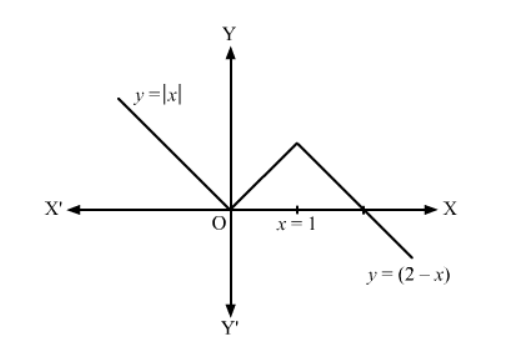# Does there exist a function which is continuos everywhere but not differentiable at exactly two points? Justify your answer ?

Question:

Does there exist a function which is continuos everywhere but not differentiable at exactly two points? Justify your answer ?

Solution:

\$y=\left\{\begin{array}{lc}|x| & -\inftyIt can be seen from the above graph that, the given function is continuos everywhere but not differentiable at exactly two points which are 0 and 1.